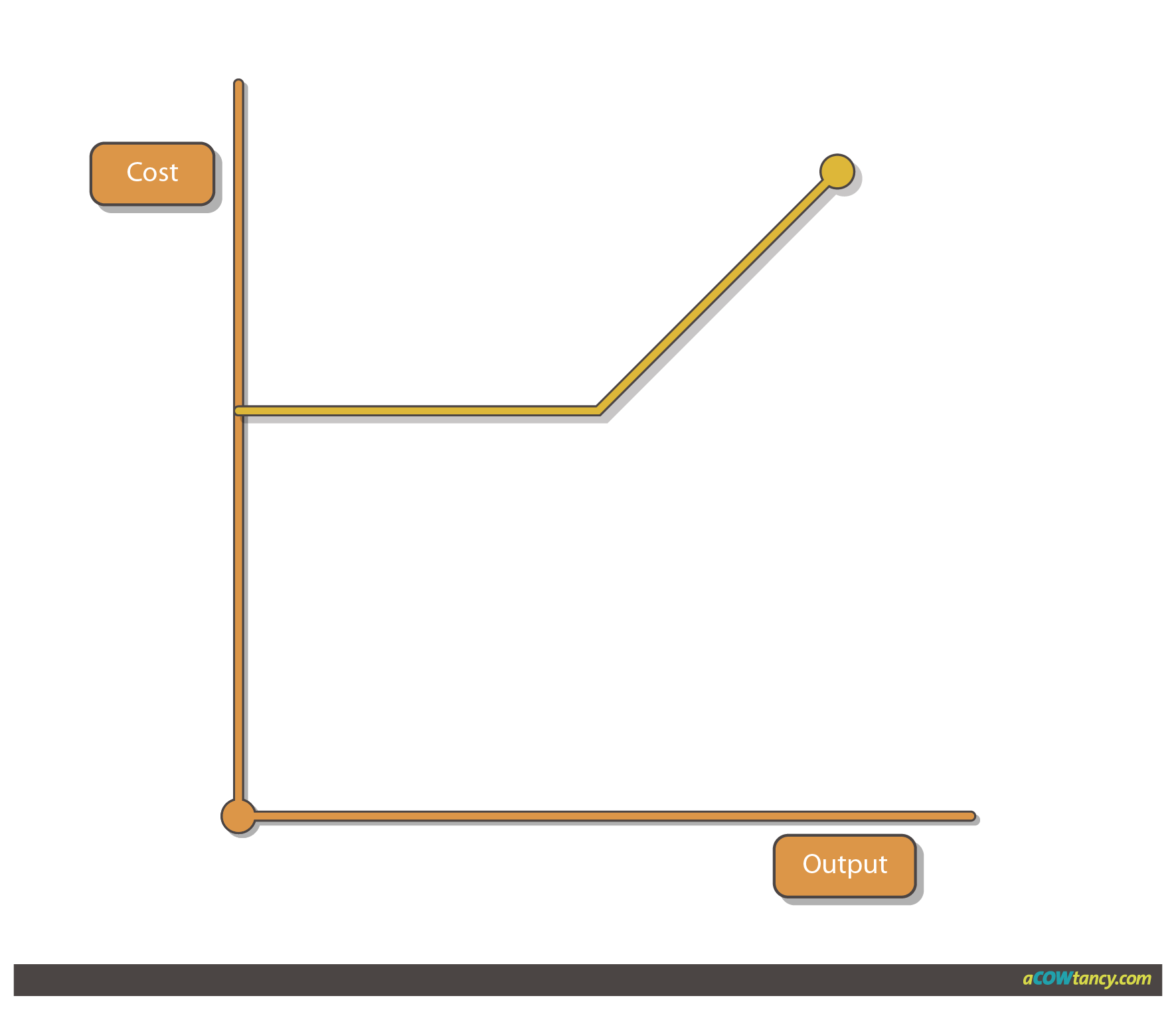MAF2
Syllabus A. Management Information A3. Cost Classification

# ACCA MA F2 Syllabus A. Management Information - A3g. Types of cost behaviour - Notes6 / 9

### Syllabus A3g)

Describe and illustrate, graphically, different types of cost behaviour.

### Types of Cost Behaviour

Costs can be classified according to the way that they behave within different levels of activity.

Cost behaviour tends to classify costs as:

• Variable cost

• Fixed cost

• Stepped fixed cost

• Semi-variable cost

### Variable Cost

A variable cost varies directly with output. (The more you make the more the cost - IN TOTAL)

However, the variable cost PER UNIT remains constant.

Direct costs are variable costs (Eg Raw materials, Labour and direct Overheads)

#### Graph 1: Total variable costs

Variable costs in total change in direct proportion to the level of activity.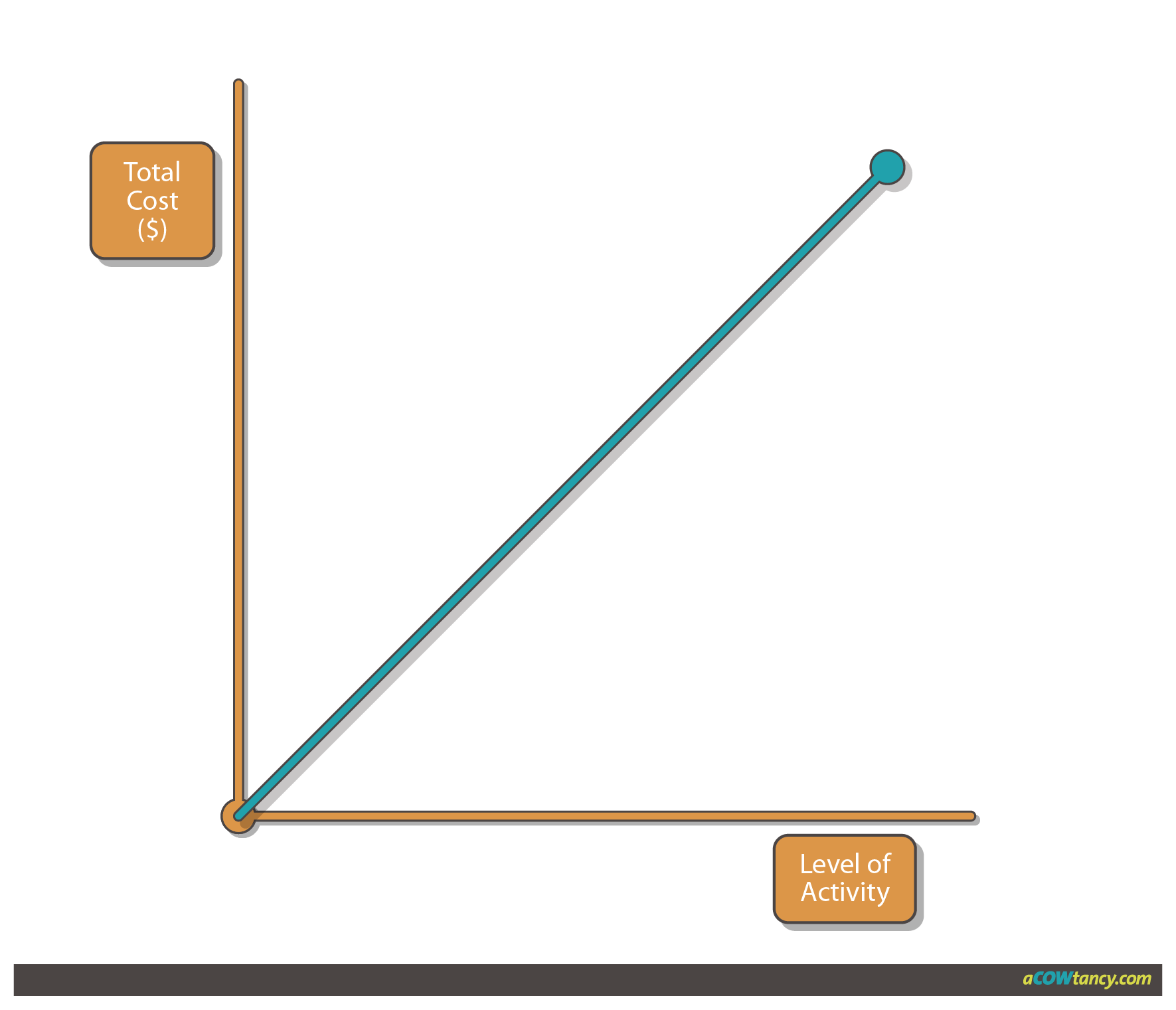#### Graph 2: Variable cost per unit

The cost per unit of variable costs remains constant.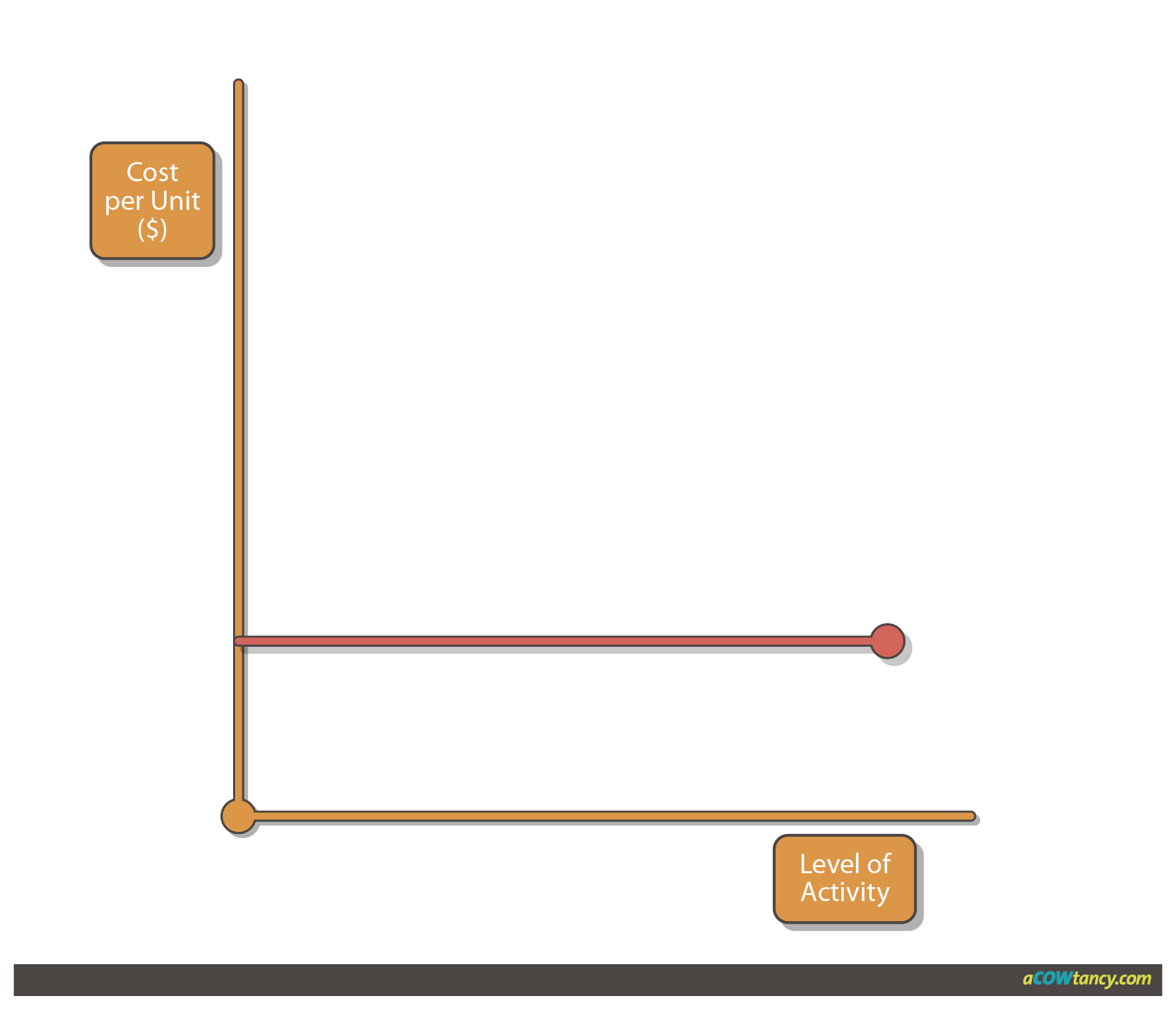### Fixed Costs

A Fixed cost (within certain activity levels) remains constant (In total)

However that also means the more the output - the Fixed Cost PER UNIT will fall

Eg Salaries, Rent,  Straight line depreciation

#### Graph 3: Total Fixed Costs

Total fixed costs remain constant over a given level of activity.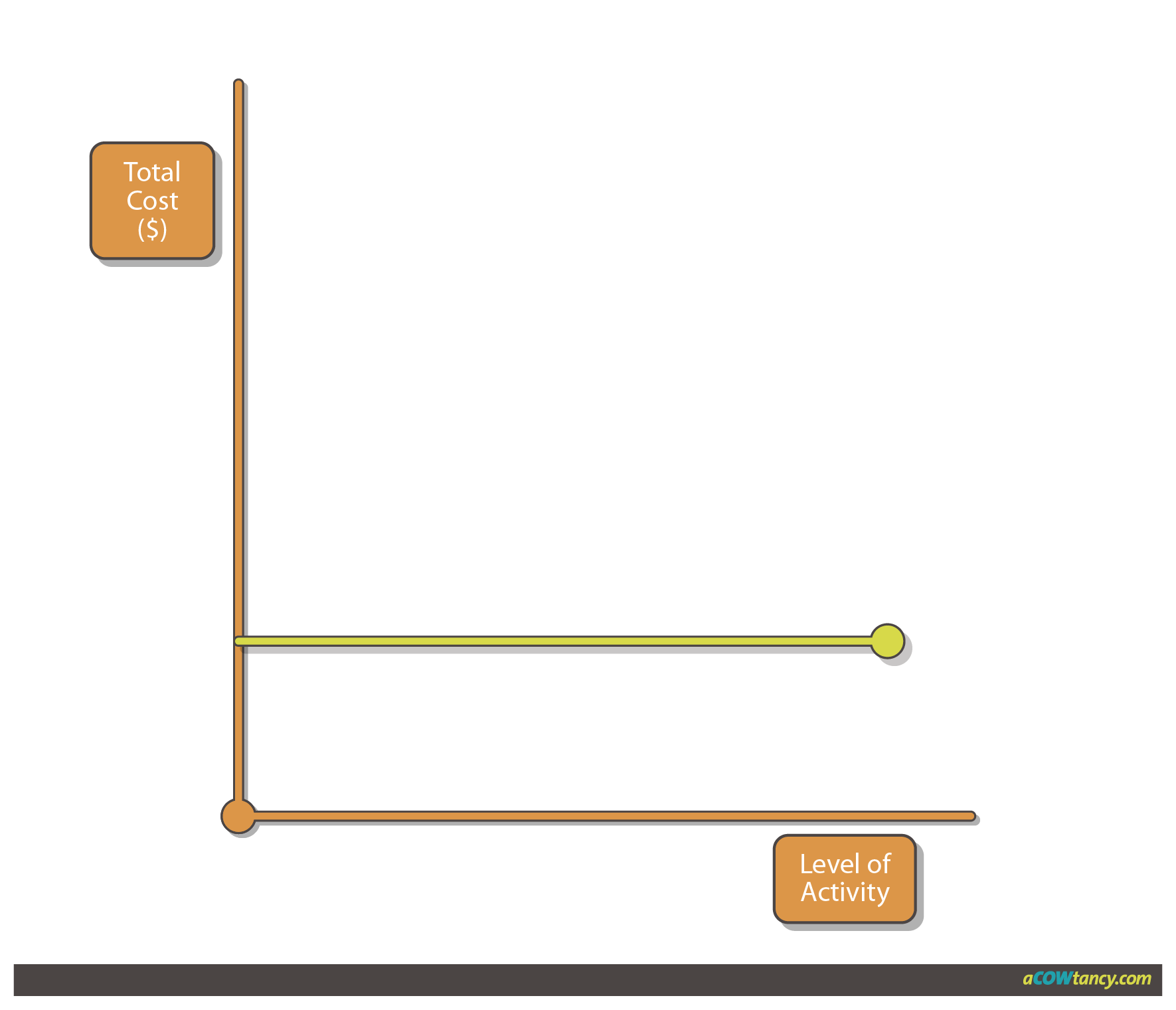#### Graph 4: Fixed Cost per unit

The fixed cost per unit falls as the level of activity increases but never reaches zero.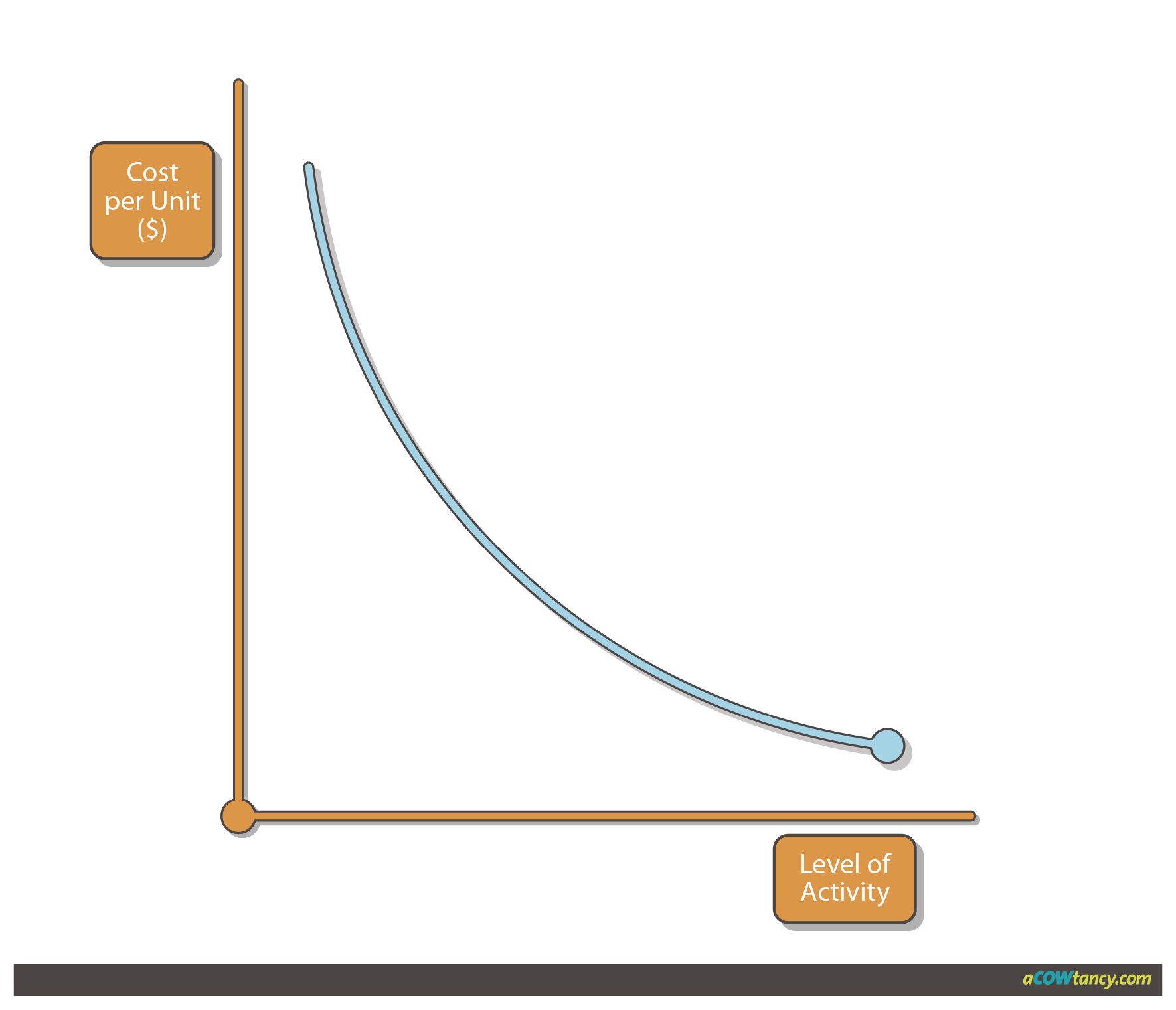### Stepped Fixed Costs

A stepped fixed cost is only fixed within certain levels of activity.

The depreciation of a machine may be fixed if production remains below 1,000 units per month. If production exceeds 1,000 units, a second machine may be required, and the cost of depreciation (on two machines) would go up a step.

Other stepped fixed costs include rent of warehouse (more space required if activity increases) and supervisors’ wages (more supervisors required if number of employees increase).

#### Graph 5: Stepped Fixed Costs

Fixed costs increase in steps as activity level increases beyond a certain limit.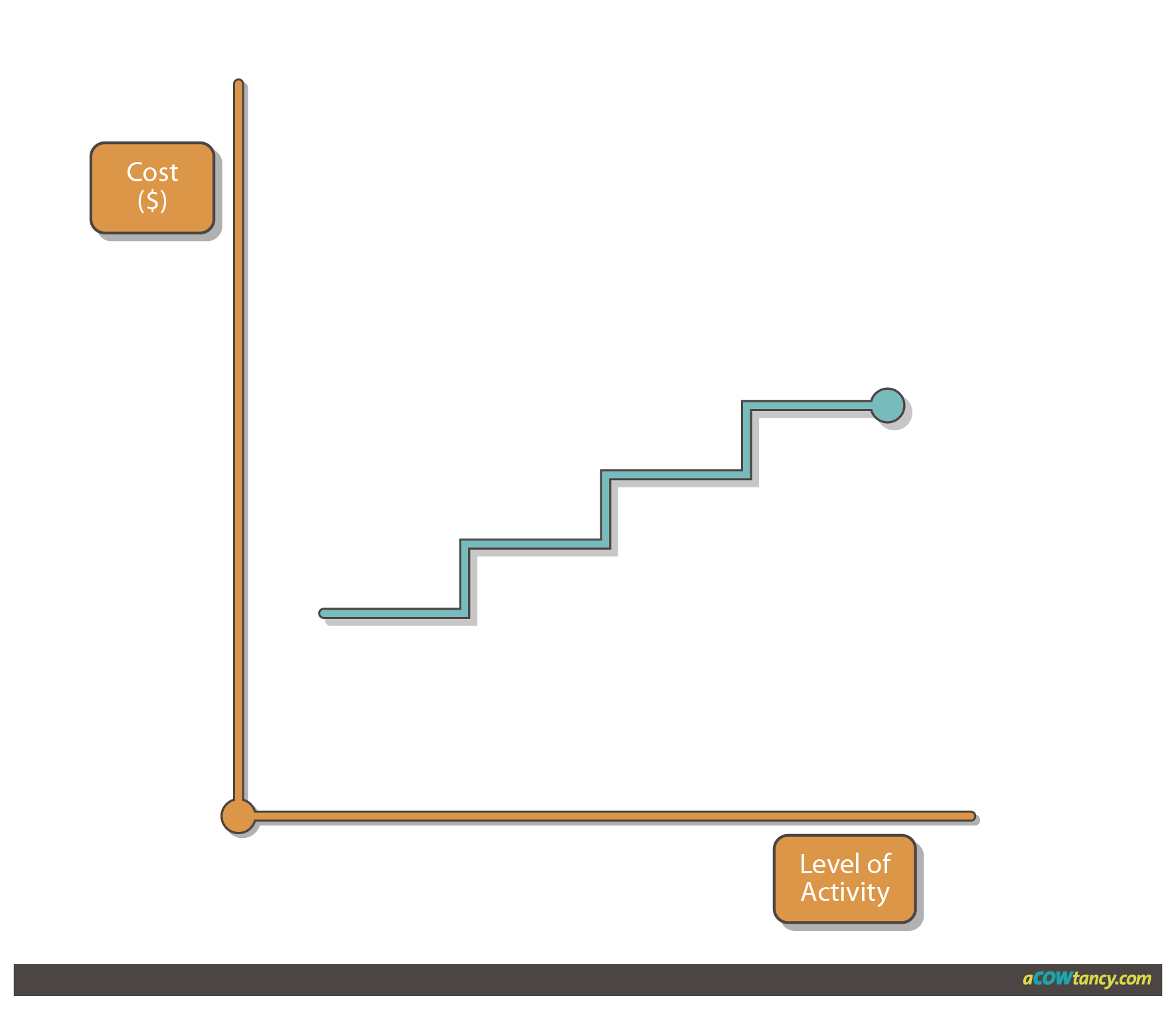### Semi-variable Costs (semi-fixed/mixed)

Semi-variable costs contain both fixed and variable components and are therefore partly affected by changes in the level of activity.

Examples of semi-variable costs includes

• Electricity and gas bills

Fixed cost = standing charge
Variable cost = electricity / gas used

• Salesman's salary

Fixed cost = basic salary
Variable cost = commission on sales made

• Costs of running a car

Fixed cost = road tax, insurance
Variable costs = petrol, oil, repairs

#### Graph 6: Total semi-variable costs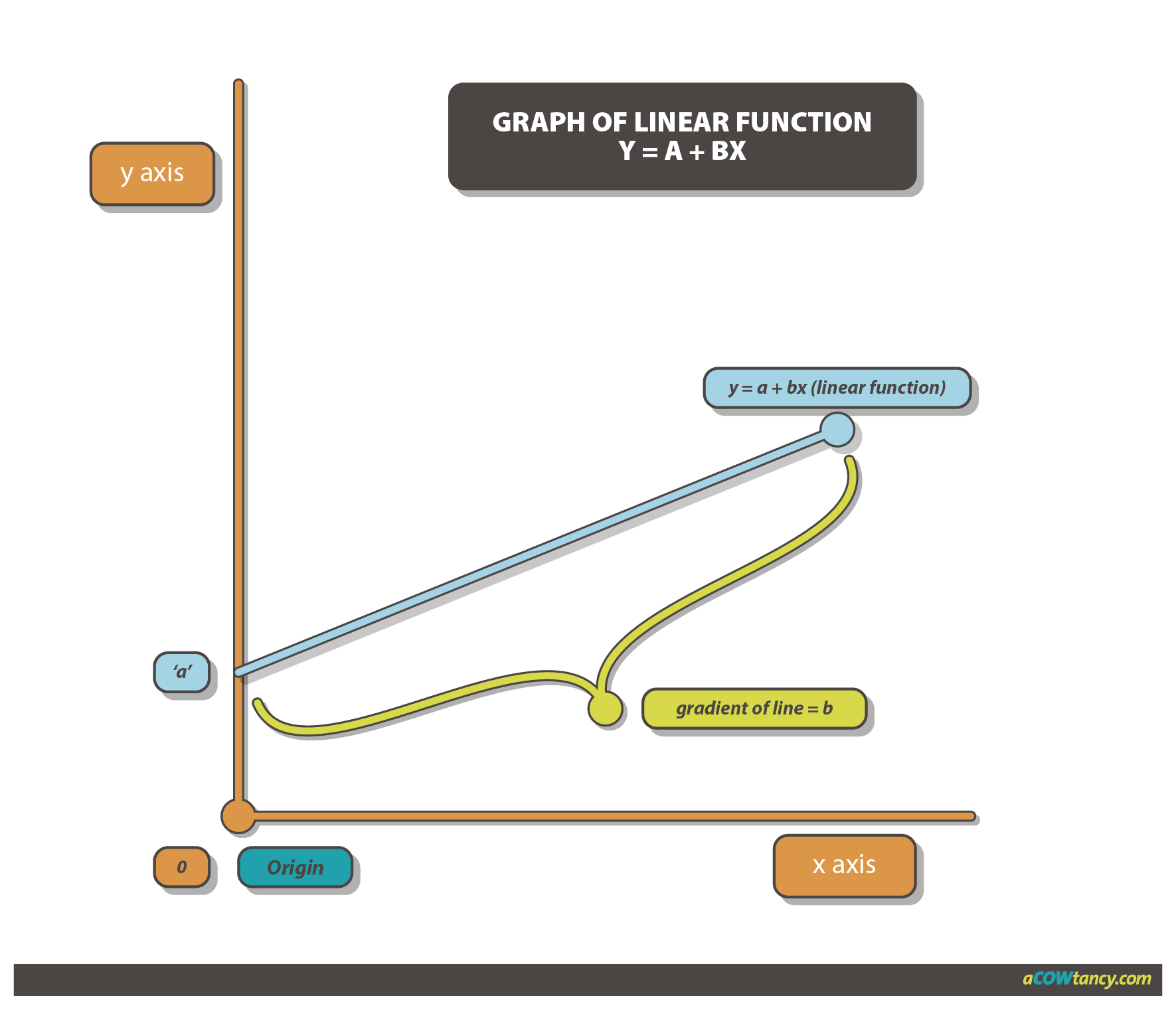#### Graph 7: Semi-variable costs per unit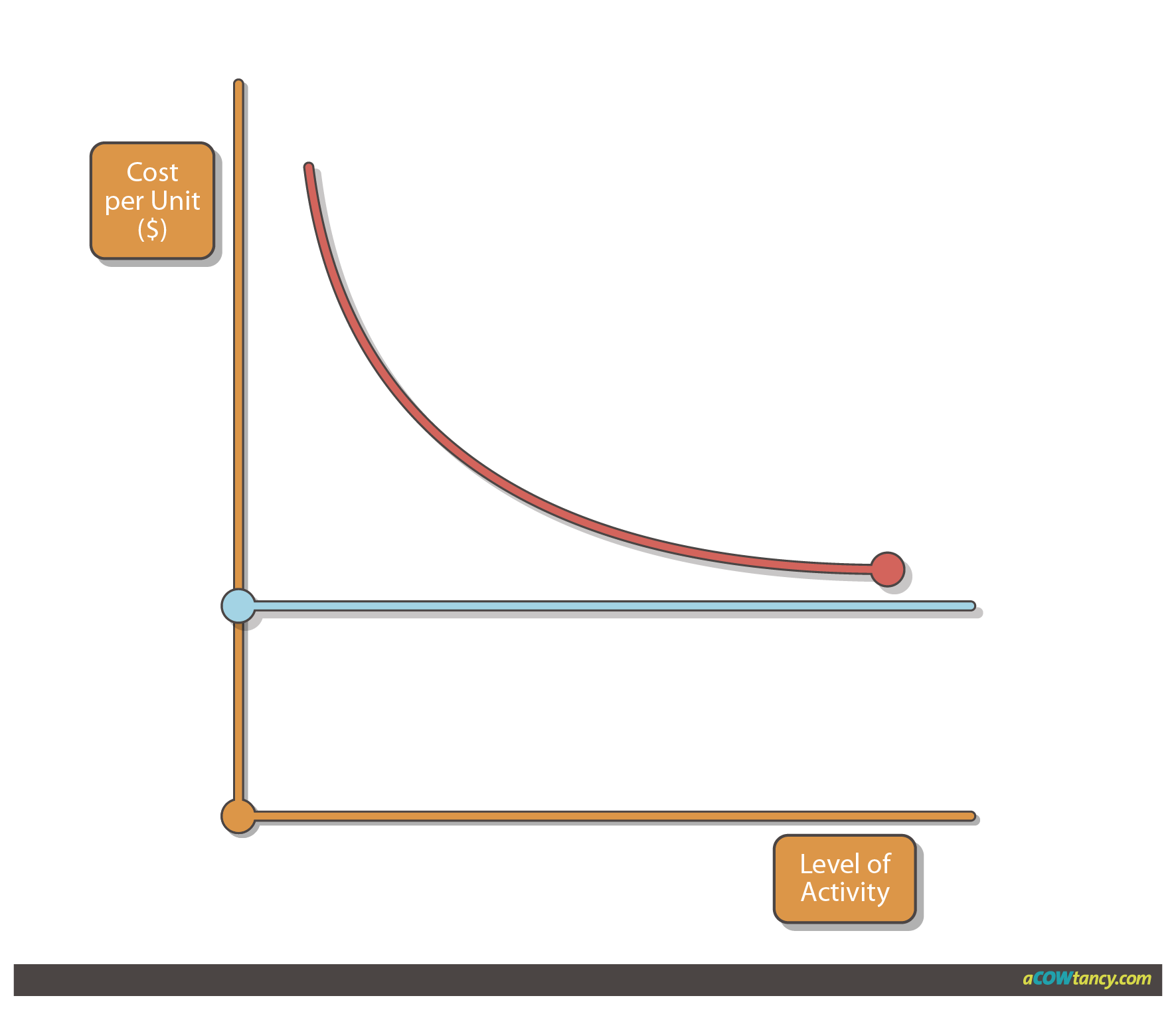#### Graph 8: Other cost behaviour patterns

This graph represents a cost which is variable with output, subject to a minimum (fixed) charge.